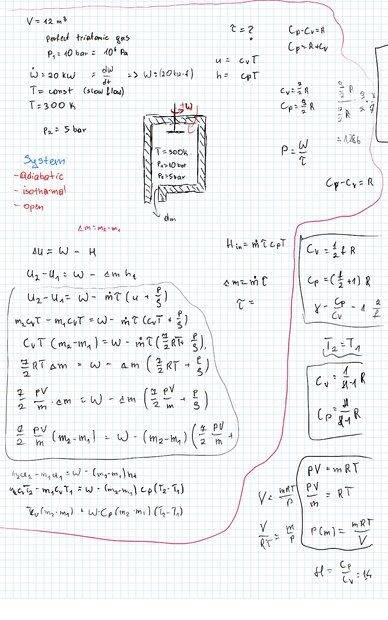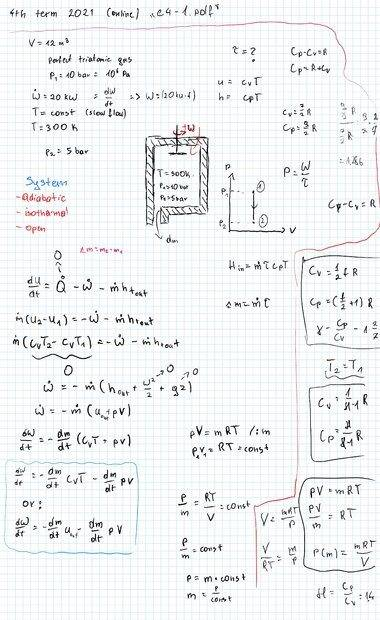# Academic First Law Of Thermodynamics Problem

Kolin101
Homework Statement:
An insulated tank of volume 12 m3 and with a valve was filled with perfect triatomic gas at pressure 10 bar. Initially the valve was closed. A paddle wheel was mounted inside the tank and was run by 20 kW engine. The paddle wheel was turn on and at the same time the exit valve was opened. The gas temperature was constant trough the whole process (due to very slow flow) and equal to 300 K.
Calculate the time needed to decrease the pressure in the tank to 5 bar.
Relevant Equations:
dU=dQ-dW-dmh
Hi there. I have a problem solving above problem. How can I move on with my solution? It seems to me that I have proper approach but I'm just stuck with the energy equation ;/

Part of solution below:Mentor
This involves application of the open-system version of the 1st law of thermodynamics, and, for this particular problem, should be formulated as a time-dependent differential equation (rather than using finite differences): $$\frac{d(nu)}{dt}=-\dot{W}+h\frac{dn}{dt}=-\dot{W}+u\frac{dn}{dt}+RT\frac{dn}{dt}$$For constant temperature, this reduces to: $$0=-\dot{W}+V\frac{dP}{dt}$$

•Kolin101
Kolin101
Thank You a lot, I've tried also this way but with no results. But to be sure, what for does the "n" stands for in your first equation?

Mentor
Thank You a lot, I've tried also this way but with no results. But to be sure, what for does the "n" stands for in your first equation?
Number of moles in tank at time t.

•Kolin101
Kolin101
ok I've figured out how would it look like in "my style solution" but I still have some questions. Why does the $$u\frac{dn}{dt}$$
term disappears? when i rewrote it using mass instead of moles, the term
$$\frac{dm}{dt}CvT$$ seems to have a non zero value (there is a mass flowing out, that caries out internal energy at T=300K) and unfortunately Cv is not known either ;/

(new solution attached below the quote)
This involves application of the open-system version of the 1st law of thermodynamics, and, for this particular problem, should be formulated as a time-dependent differential equation (rather than using finite differences): $$\frac{d(nu)}{dt}=-\dot{W}+h\frac{dn}{dt}=-\dot{W}+u\frac{dn}{dt}+RT\frac{dn}{dt}$$For constant temperature, this reduces to: $$0=-\dot{W}+V\frac{dP}{dt}$$Mentor
ok I've figured out how would it look like in "my style solution" but I still have some questions. Why does the $$u\frac{dn}{dt}$$
term disappears?
It cancels from both sides of the equation. What is the derivative of un with respect to t? On the rhs, h=u+Pv.